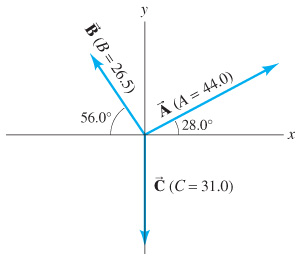# Problem: For the vectors given in the figure, determine(a) A - B + C(b) A + B - C(c) C - A - B

###### FREE Expert Solution
86% (285 ratings)
###### Problem Details

For the vectors given in the figure, determine
(a) A - B + C
(b) A + B - C
(c) C - A - B